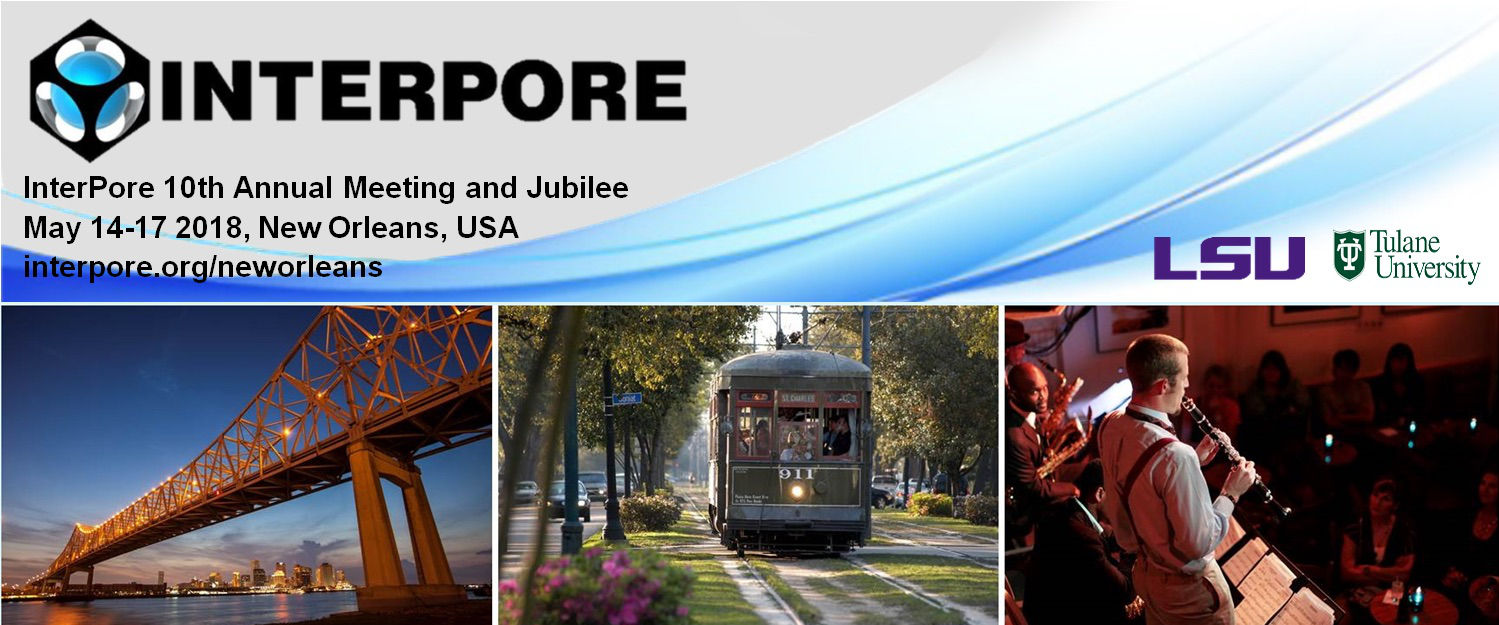#InterPore2018 New Orleans

14-17 May 2018
New Orleans
US/Central timezone

## A Linear Domain Decomposition Method for Unsaturated Flow in Porous Media

16 May 2018, 18:30
15m
New Orleans

#### New Orleans

Poster MS 2.13: Advances in numerical modelling of multiphase flow and transport in fractured porous media

### Speaker

Koondanibha Mitra (Eindhoven University of Technology)

### Description

The Richards equation is a commonly used model for unsaturated flow through porous media. Using the Darcy law in the mass balance equation, and bringing the resulting equation to a dimensionless form one obtains:
\begin{equation}
\phi\partial_t S=\nabla\cdot\left(\frac{K}{\mu} k_r(S)\nabla (p -\rho g z)\right)+f(S,\vec{x}).
\end{equation}
Here $\phi$ is the porosity of the medium; $z$ is the height; $\rho$, $\mu$ are the density and the viscosity of water respectively. The nonlinear function $k_r(S)$ (Relative permeability) is determined based on experiments and $K$ (Absolute permeability) can vary in space. Two unknowns are involved: $S$, the saturation and $p$, the pressure of water. Commonly, it is assumed that these are related by a nonlinear relationship which is again determined based on experiments:

\begin{equation}
-p=P_c(S).
\end{equation}
The exact form of these nonlinearities depend on the medium. Most realistic problems involve heterogeneous media. Example in this sense are layered or fractured oil and gas reservoirs. In this case, the functions themselves are changing depending on the location inside the medium. In particular, if the adjacent blocks are separated by a fracture then this poses difficulties for the numerical simulation as generally different spatial discretization, models and pre-conditioners are used inside the fractures than in the blocks.

In this work we consider a medium consisting of $N$ homogeneous sub-domains and propose a non-overlapping domain decomposition scheme that couples the models in the whole domain. The scheme involves linear iterations [Pop et al (2004), List and Radu (2016)]. Within one iteration the sub-domains are completely decoupled and are solved independently [Seus et al. (2017)]. After analyzing its convergence, we show some numerical results confirming the theoretical findings and discuss different implementation aspects.

### References

Seus, D., Mitra, K., Pop, I. S., Radu, F. A., & Rohde, C. (2017). A linear domain decomposition method for partially saturated flow in porous media. arXiv preprint arXiv:1708.03224.

Pop, I. S., Radu, F.A., & Knabner, P. (2004). Mixed finite elements for the Richards’ equation: linearization procedure. Journal of computational and applied mathematics, 168(1), 365-373.

List, F., & Radu, F. A. (2016). A study on iterative methods for solving Richards equation. Computational Geosciences, 20(2), 341-353.